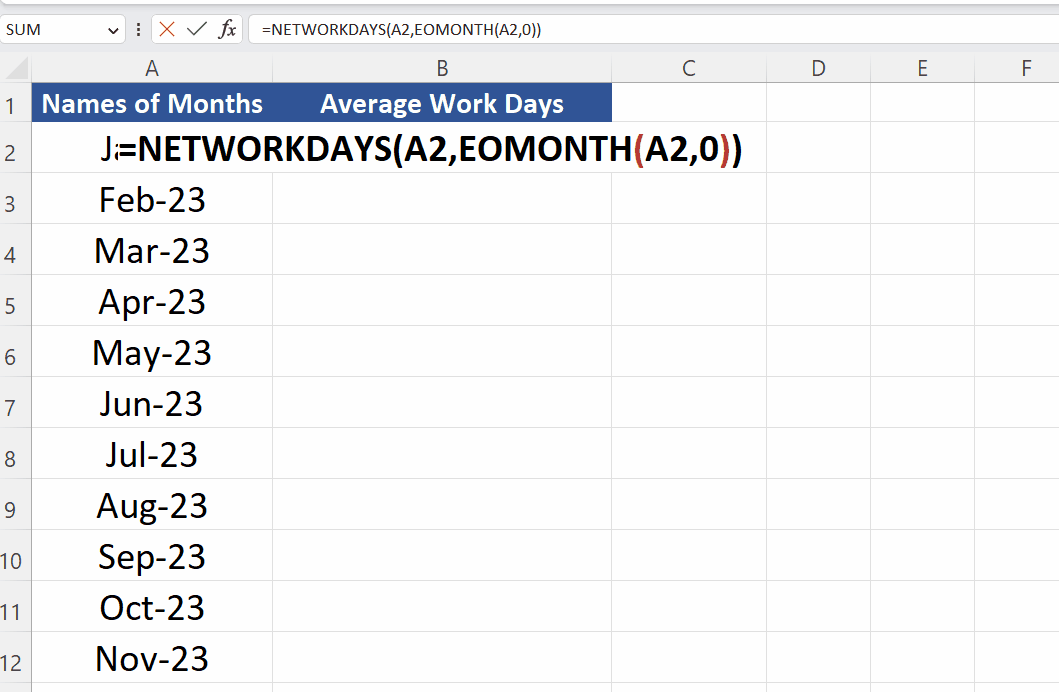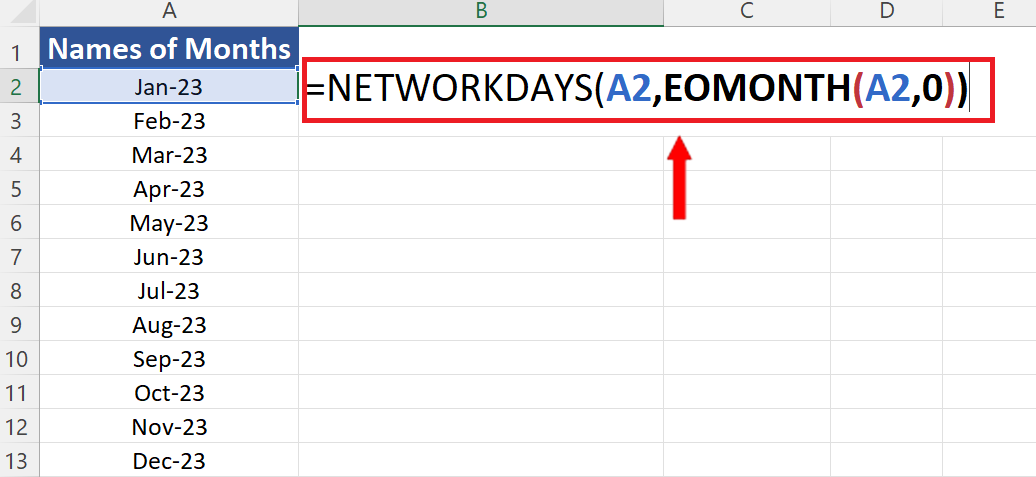# How to calculate average weekdays of a month in Excel

In this tutorial we’ll calculate the average working days of months of the current year by using a combination of functions, NETWORKDAYS & EOMONTH. We have the dataset shown below, in which we have the 1st day of each month from January to December 2023. However, we have formatted the dates in such a way that Excel shows only the month name and year numbers as shown above.

Let’s see the syntax of both functions first i.e., NETWORKDAYS and EOMONTH.

## Syntax of NETWORKDAYS

The syntax for the NETWORKDAYS function in Excel is as follows:

NETWORKDAYS(start_date, end_date, [holidays])

where:

start_date is the start date for the calculation.

end_date is the end date for the calculation.

[holidays] is an optional argument that represents a range of cells that contain the dates of holidays or non-working days.

For example, to calculate the number of working days between January 1st, 2023 and February 15th, 2023, excluding weekends and a list of holidays stored in cells B2:B5, the following formula could be used:

=NETWORKDAYS(“1/1/2023”, “2/15/2023”, B2:B5)

## Syntax of EOMONTH

The syntax for the EOMONTH function in Excel is as follows:

EOMONTH(start_date, months)

where:

start_date is the start date for the calculation.

months is the number of months to add to the start date. A positive value will add months to the start date, while a negative value will subtract months from the start date.

For example, to find the end of the month for January 15th, 2023, the following formula could be used:

=EOMONTH(“1/15/2023”, 0)

This would return the date for the end of the month for January 15th, 2023, which would be January 31st, 2023.

In our case, by using the combination of NETWORKDAYS and EOMONTH we can easily find the average working days in a month. The NETWORKDAYS function returns us the total number of days between start and end dates excluding the weekends and the special holidays. The list of custom holidays can also be used if it is listed in a range in Excel.

EOMONTH is used in conjunction with NETWORKDAYS to get the last date of the each month. Let’s see how we can calculate the working days by implementing the above explained functions.While working on a project planning, you might need to calculate average workdays in a month in Excel when you want to determine the average number of workdays in a month for a given period. For example, you might want to know the average number of workdays in a month over the past year for planning purposes, or you might want to estimate how much time it will take to complete a project based on the average number of workdays in a month.

### Step 1 – Create a list of months from dates– Write the date 01/01/2023 in the first cell of your dataset.
– Then press CTRL+1 to open the Format Cells dialog box.
– Now choose the Custom option from the Category list.
– Choose mmm-yy in the Type category.
– This will convert the dates to Month name and Year number only as shown above.

### Step 2 – Implement the formula– Now it’s time to implement the formula i.e.,
=NETWORKDAYS(A2,EOMONTH(A2,0))
– In this formula A2 contains the 1st date of the month Jan 2023 and EOMONTH returns the last day of Jan 2023. The 0 in the EOMONTH tells the function to return the last date of the month mentioned in the first parameter.

### Step 3 – Implement the formula– After writing the appropriate formula, press the enter button. This will implement the formula in the cell.
– Now double click the fill handle to implement the same formula for the whole range of data as shown above.